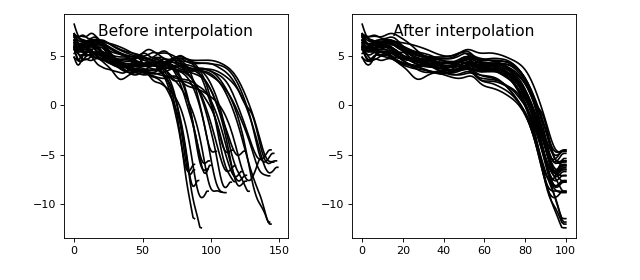# Data processing basics¶

The examples below detail pre-processing functionality in spm1d.util.

Warning

spm1d currently offers only very basic data processing functions.

• Please consider using other software tools like SciPy and Visual3D for data processing.

## Interpolation¶

`./spm1d/examples/processing/ex_interpolate.py`

Linear interpolation to n points:

```>>> y0 = np.random.rand(51)
>>> y1 = np.random.rand(87)
>>> y2 = np.random.rand(68)
>>> Y  = [y0, y1, y2]
```
```>>> Y  = spm1d.util.interp(Y, Q=101)
```## Smoothing¶

`./spm1d/examples/processing/ex_smooth.py`

Convolution with a Gaussian kernel having a given FWHM (full-width at half-maximum):

```>>> Y_smoothed = spm1d.util.smooth(Y0, fwhm=5.0)
```